# Multiplying Fractions

Of all the operations involving fractions, multiplying is probably the easiest.

All you need to do is to change any mixed numbers into improper fractions, multiply the numerators, multiply the denominators and then simplify the result.

Lets look at an example: ½ x ¼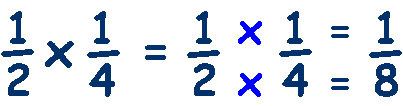Lets try another: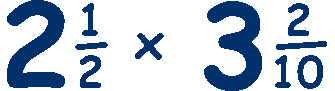Change mixed numbers into improper fractions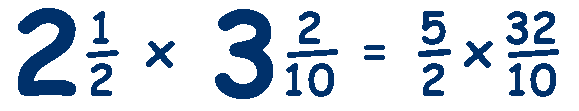Multiply the numerators and denominatorsAnd simplify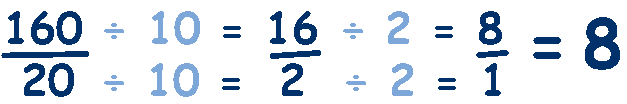When multiplying, you can simplify before calculating if any numerator and denominator have common factorsThe 5 and 10 have a common factor of 5, divide both by 5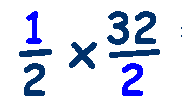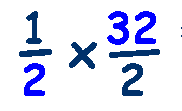The 2 and 32 have a common factor of 2, divide both by 2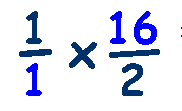Now the 16 and the 2 have a common factor of 2, divide by 2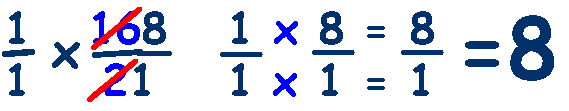This method of simplifying the fractions before doing any calculations can greatly reduce the time and effort required and you will find that most questions can be simplified first. Look at another example: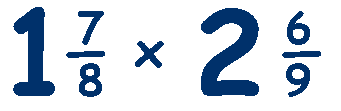Change mixed numbers into improper fractionsSimplify (cancel out) 15 and 9 by dividing by 3Simplify (cancel out) 24 and 8 by dividing by 8Simplify (cancel out) 3 and 3 by dividing by 3Multiply the numerators and denominatorsThis method is worth persevering with as once your child gets the hang of it, it can really speed up calculations and save time in the exam.

Try this Fraction Multiplication Worksheet

and

Multiplying Fractions Quiz
from Dr. Mike